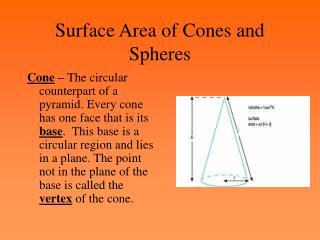DownloadDownload PresentationSurface Area of Cones and Spheres

# Surface Area of Cones and Spheres

Télécharger la présentation## Surface Area of Cones and Spheres

- - - - - - - - - - - - - - - - - - - - - - - - - - - E N D - - - - - - - - - - - - - - - - - - - - - - - - - - -
##### Presentation Transcript

1. Surface Area of Cones and Spheres Cone – The circular counterpart of a pyramid. Every cone has one face that is its base. This base is a circular region and lies in a plane. The point not in the plane of the base is called the vertex of the cone.

2. Surface Area of Cones and Spheres Altitude – The perpendicular line segment connecting the apex of the cone to the plane of its base. The length of this segment is frequently termed the height of the cone. Axis – The segment connecting the center of one base to the apex. If the axis is perpendicular to the plane of the base, the cone is right. If the axis is not perpendicular, the cone is oblique.

3. Surface Area of Cones and Spheres Lateral Area of a Cone • If a right circular cone has a lateral area of L square units, a slant height of l units, and the radius of base is r units, then L = *r*l

4. Surface Area of Cones and Spheres Surface Area of a Cone • If a right circular cone has a surface area of T square units, a slant height of l units, and the radius of the base is r units, then T = *r*l + *r2

5. Example 6-1e A hat for a child’s birthday party has a conical shape with an altitude of 9 inches and a diameter of 5 inches. Find the lateral area of the birthday hat. Answer: 73.4 in2

6. Example 6-2b Find the surface area of the cone. Round to the nearest tenth. Answer: about 63.6 cm2

7. Surface Area of Cones and Spheres Great Circle – When a plane intersects a sphere so that the intersection contains the center of the sphere. The radius of the sphere and the great circle are the same length.

8. Surface Area of Cones and Spheres Hemisphere – Half of a sphere. A great circle splits a sphere into two congruent hemispheres.

9. In the figure, O is the center of the sphere, and plane U intersects the sphere in circle L. If OL3 inches and LM8 inches, find OM. Example 7-1c Answer: about 8.5 in.

10. Surface Area of Cones and Spheres Surface Area of a Sphere • If a sphere has a surface area of T square units and a radius of r units, then T = 4**r2

11. Example 7-2c a. Find the surface area of the sphere, given a great circle with an area of approximately 91.6 square centimeters. b. Find the surface area of a hemisphere with a radius of 6.4 inches. Answer: about 366.4 cm2 Answer: about 386.0 in2

12. Example 7-3c Find the surface area of a ball with a circumference of 18 inches to determine how much leather is needed to make the ball. Answer: about 103.1 in2